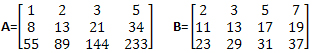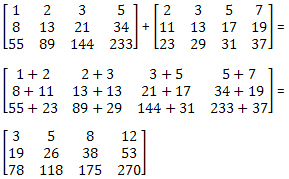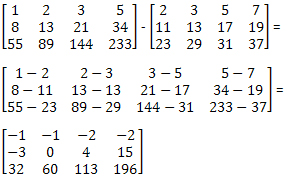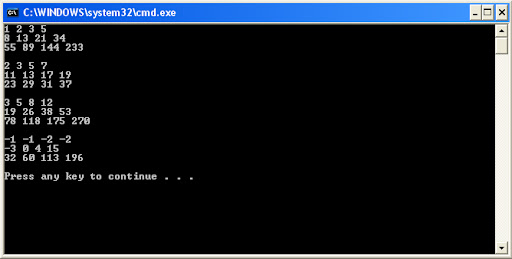## Saturday, February 16, 2013

### Basic Matrix Operations with VB.NET Part 2: Matrix Addition and Subtraction

You can add or subtract two matrices if they each have the same number of rows and columns.   Let’s take our example matrix from the previous post , A , and add it to a second matrix, B .Adding these two matrices is as simple as adding each element in A to its corresponding element in B .   For example, the first row first column value in A is 1. The first row first column value in B is 2. So the first row first column value in A+B is 1+2 or 3. Calculating the whole thing looks like this.Matrix subtraction works the same way, only you subtract each element in A from its corresponding element in B . Subtracting A from B looks like this.As I mentioned in the last post , you can represent a matrix in VB.NET with a multidimensional array. In the code for the example calculations above we will build four arrays, A,   B, C and D. Arrays A and B correspond to matrices A and B . Array C will contain the sum of matrices A and B , and D will contain the difference between A and B .

Let’s begin by building A and B and populating the values.

Sub Main()
Dim A(2, 3) As Integer
A(0, 0) = 1
A(0, 1) = 2
A(0, 2) = 3
A(0, 3) = 5
A(1, 0) = 8
A(1, 1) = 13
A(1, 2) = 21
A(1, 3) = 34
A(2, 0) = 55
A(2, 1) = 89
A(2, 2) = 144
A(2, 3) = 233

Dim B(2, 3) As Integer
B(0, 0) = 2
B(0, 1) = 3
B(0, 2) = 5
B(0, 3) = 7
B(1, 0) = 11
B(1, 1) = 13
B(1, 2) = 17
B(1, 3) = 19
B(2, 0) = 23
B(2, 1) = 29
B(2, 2) = 31
B(2, 3) = 37

Next, build C and D.

Dim C(2, 3) As Integer
Dim D(2, 3) As Integer

We populate the values for C and D with nested FOR NEXT loops. The outer loop, x, corresponds to the rows in each array and the inner loop, y, corresponds to the columns in each array.   Populate C by adding the corresponding elements x,y from A and B. Populate D by subtracting the corresponding elements x,y from A and B.

Dim x, y As Integer
For x = 0 To 2
For y = 0 To 3
C(x, y) = A(x, y) + B(x, y)
D(x, y) = A(x, y) - B(x, y)
Next
Next

Now run the nested loops again to for each array and write them to the screen.

For x = 0 To 2
For y = 0 To 3
Console.Write(A(x, y))
Console.Write(Space(1))
Next
Console.WriteLine ()
Next
Console.WriteLine()

For x = 0 To 2
For y = 0 To 3
Console.Write(B(x, y))
Console.Write(Space(1))
Next
Console.WriteLine()
Next
Console.WriteLine()

For x = 0 To 2
For y = 0 To 3
Console.Write(C(x, y))
Console.Write(Space(1))
Next
Console.WriteLine()

Next
Console.WriteLine()

For x = 0 To 2
For y = 0 To 3
Console.Write(D(x, y))
Console.Write(Space(1))
Next
Console.WriteLine()
Next
Console.WriteLine()

End Sub

And here is our output.Next time we'll look at matrix multiplication.